### This deep dive into latches, CyclicBarriers, and phasers uses a stencil algorithm to demonstrate how all of them work and how to reduce synchronization contention.

· Java Zone · Tutorial
Save
14.91K Views

i recently came across java phasers while reviewing different constructs for inter-thread communication. phasers are associated to the concepts of fuzzy barriers and point-to-point synchronization .

as for this post, i will borrow the example of the one-dimensional stencil to explain very intuitively the motivation behind the concept of phasers.

the code corresponding to all the examples in this post can be found on this repository .

## one-dimensional stencil

in order to justify the need for phasers, we will start by demonstrating the use of other synchronization constructs to solve the computational problem of the one-dimensional stencil.

given a one-dimensional array a with n elements, the value of each element is calculated according to this function:in other words, the value of the first and last elements is fixed (these values do not need to be the same though), whereas the value of each of the remaining n-2 elements is the average of the value of its neighbors. this is a recursive process: as the value of its neighbors change, each element needs to re-compute its own value. ideally, after a few iterations, the value of all the elements should converge and the recursive iterations stop.

just a brief digression for those curious about physics: the aforementioned wikipedia link says that “stencil codes are most commonly found in the codes of computer simulations.” no wonder! you can picture the one-dimensional stencil as a metal bar connected to a heat source by its sides. the temperature of the sides remains constant whereas the temperature along the bar changes over time until reaching thermal equilibrium.

to get some intuition about the stencil problem, let’s work through an example. to make it simple, consider an array of length 4 whose first and last elements have a fixed value 1. the remaining elements have an initial value of 2. therefore, the initial array is [1,2,2,1]. after the first iteration, the array becomes [1,1.5,1.5,1]. the following diagram shows how the values change on each iteration and how, eventually, the values converge to 1.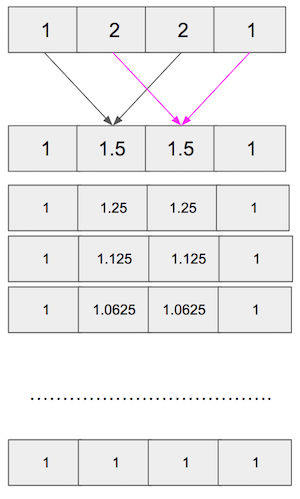stencil algorithm

implementation detail : in order to avoid creating a new array on each iteration, the implementation of the algorithm can use just two arrays: one containing the values at the beginning of the iteration and another containing the resulting values at the end. then, for the next iteration, the arrays are flipped. this diagram illustrates the process: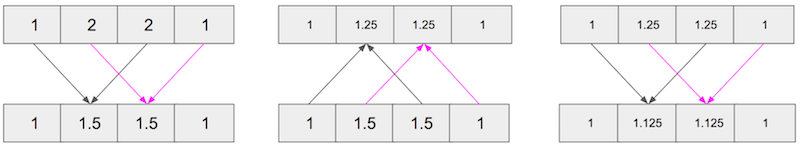stencil algorithm simplified

and the snippet below is the sequential implementation of the algorithm (the code snippets presented in this post are written in scala, although any java developer can easily read them):

/**
* sequential version
* @param iter number of iterations
* @param arr array with fixed boundary values. the other values are calculated as the average value of their neighbours
* @return array with the final values after completing all iterations
*/
def seq(iter: int, arr: array[double]): array[double] = {
//defensive copy to keep arr from being mutated outside this method
var myval = arr.clone()
var mynew = arr.clone()

for(j <- (1 to iter)) {
for (i <- (1 until arr.length-1)) {
mynew(i) = (myval(i - 1) + myval(i + 1)) / 2.0
}
val temp = mynew
mynew = myval
myval = temp
}
myval
}

## parallel implementations

working on top of the sequential solution, this section describes other approaches to solve the problem using different parallel-programming constructs: latch, cyclic barrier, and phasers .

### latch

according to countdownlatch , a latch is “a synchronization aid that allows one or more threads to wait until a set of operations being performed in other threads completes.”

the solution to the stencil problem with latches involves the following steps:

• create a new latch for each iteration with a count equal to n-2.
• create n-2 tasks to be executed in parallel by threads of a dedicated execution context while the main thread waits until all tasks are completed; each task calculates the value of one element of the array.
• once a task is finished, the last action of the executing thread is to let the main thread know that the task is completed.
• when all tasks are terminated, the main thread resumes its activity by flipping the old and new arrays and starting a new iteration.

it is worth noting that:

• the communication through the latch happens between each of the threads of the dedicated execution context and the main thread.
• the latch represents a barrier to the execution of the main thread only.

the following diagram represents 2 iterations of the process just described: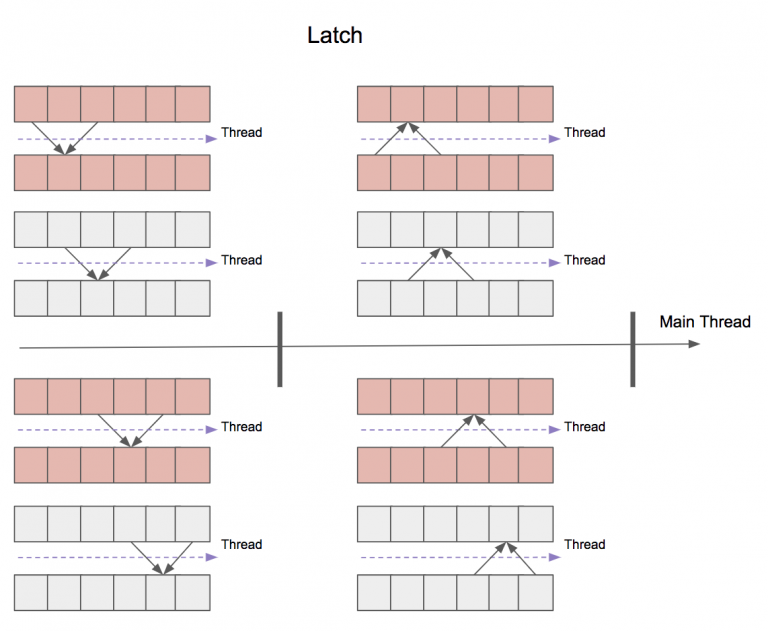latch

### cyclic barrier

from the definition of cyclicbarrier , a cyclic barrier is “a synchronization aid that allows a set of threads to all wait for each other to reach a common barrier point.”

here are the main characteristics of this solution:

• each of the n-2 tasks calculates the value of one element of the array from beginning to end — that is, through all the iterations.
• these tasks are not independent, as the values of the neighbors that each task needs to calculate its own value are calculated by other tasks.
• therefore, before moving onto a new iteration, each task needs to wait for all others to complete.

this problem seems a good match for a cyclic barrier and comprises the following steps:

• create a cyclic barrier with n-2 parties.
• create n-2 tasks to be executed in parallel by threads of a dedicated execution context. each task calculates the value of one element of the array through all iterations.
• at the end of each iteration, each thread waits at the barrier until all other threads reach it.
• finally, the arrays are flipped and a new iteration begins.

the main features of this algorithm are:

• the communication through the barrier happens among all threads of the dedicated execution context.
• the cyclic barrier represents a common barrier for all threads.

the following diagram represents two iterations of the process: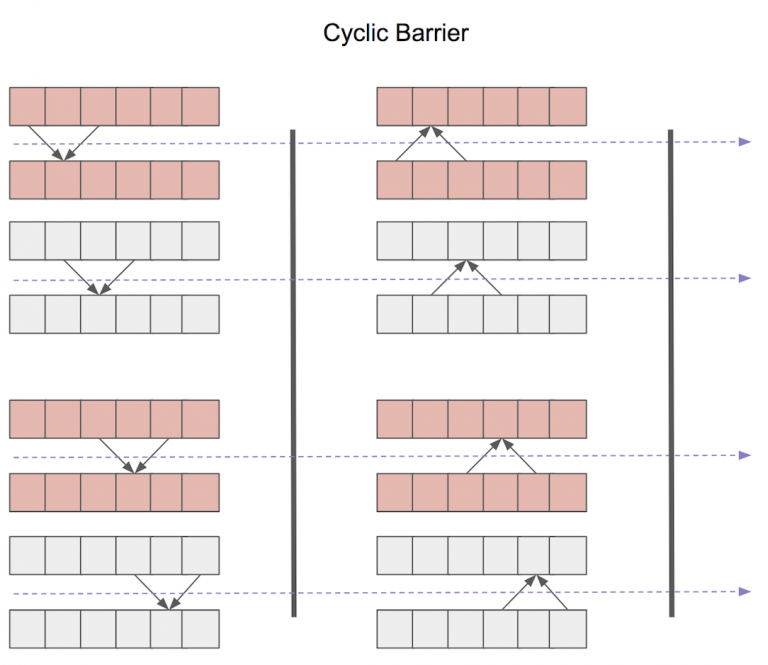cyclic barrier

### phasers

phasers allow you to reduce the synchronization contention cost when there is a large number of parties. in order to understand this, let’s revisit the cyclic barrier example: we said that “before moving on to a new iteration, each task needs to wait for all others to complete,” and as a consequence, there is a common barrier for all threads.

well, the above statement is not completely true. strictly speaking, each task needs to wait only for the adjacent tasks that compute the value of its neighbors. therefore, the situation is better described by the following diagram, where each thread shares a barrier with the adjacent ones. this fine-grained synchronization is achieved through the use of phasers. the implementation details can be found in the source code .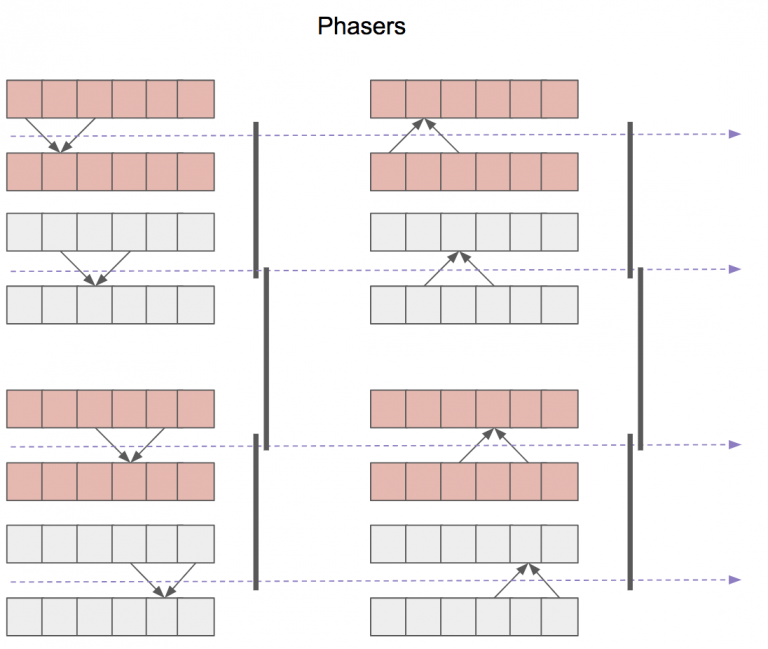phasers

### a final twist: fuzzy barriers

when dealing with cycling barriers and phasers, it was mentioned that the number of threads needed is n-2. for large arrays (think of millions of elements), this is not feasible. you will get the dreaded

an exception or error caused a run to abort: unable to create new native thread
java.lang.outofmemoryerror: unable to create new native thread
at java.util.concurrent.threadpoolexecutor.execute(threadpoolexecutor.java:1357)

and even if it were possible to create so many threads, the operating system would struggle to handle them all with a limited number of cpus.

in order to get around this issue, the elements of the array can be grouped and then create a task for each group. each task will calculate the values of all the elements of that group in a sequential fashion, and the creation and synchronization of barriers will happen at the group level.

as discussed in the previous section, the different approaches could be:

• cyclicbarrier : in order to progress to the next phase, all the elements of each group must be calculated.
• phasers : to move to the next phase, a thread only needs to wait for the elements of the adjacent groups to be calculated

let’s take a closer look at the last point. in reality, a thread only needs to wait for the first/last element of the adjacent groups to be calculated. therefore, each thread can:

• first, calculate the first and last elements of its group.
• signal to the other threads that the first/last elements are calculated (this is interpreted as the thread reaching the first point of the synchronization barrier) and, therefore, those other threads can progress to the next phase (if they are ready to do so).
• calculate the internal elements of its group (which are not depended upon by the other threads).
• wait at the second point of the barrier for the threads of the adjacent groups to reach the first point of the barrier

this is called phasers with split-phase barriers or fuzzy barriers . this capability to perform local work within the barrier is another main characteristic of phasers, together with the capability to make fine-grained synchronization possible.

the following diagram illustrates the idea of fuzzy barriers. the diagram represents a 12-element array divided in 3 groups of 4 elements. to keep things simple, we will focus on the barrier shared by the first and second groups.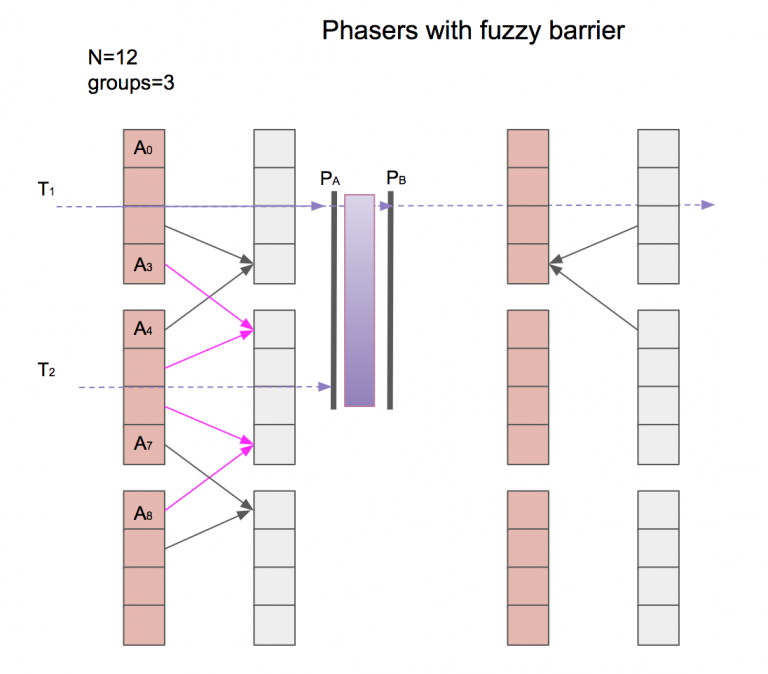fuzzy barrier

each thread t x calculates the value of all elements in that group, starting with the values on the sides.

for instance, t 1 calculates a 3 , reaches p a , and signals it out to the other threads. then, it proceeds to calculate the rest of the elements in the group and, when it is done, waits on p b until t 2 reaches p a .

when t 2 has calculated a 4 and a 7 , it reaches p a and emits the corresponding signal. at this point, t 1 can progress to phase 2 while t 2 completes phase 1 by calculating the rest of the elements of its group, and so on.

## conclusion

the journey from the latch solution to phasers has led us to reduce the synchronization contention costs by means of:

1. changing from countdownlatch to cyclicbarrier to swap the iterative actions of the algorithm:  for each iteration { for each array element { ... } } -> for each array element { for each iteration { ... } } 
2. changing from cyclicbarrier to phasers to break up the large common synchronization barrier into small individual ones
3. grouping elements into groups that are processed in parallel (while the content of each group is processed sequentially):  for each group {for each iteration { for each array element { ... } }} 
4. introducing fuzzy barriers to perform local work inside the barrier, therefore further reducing the contention

the code for all the examples discussed in this post can be found on this repository .

Topics:
java, java phasers, fuzzy barriers, synchronization, stencil algorithm, tutorial

Published at DZone with permission of Francisco Alvarez, DZone MVB.

Opinions expressed by DZone contributors are their own.